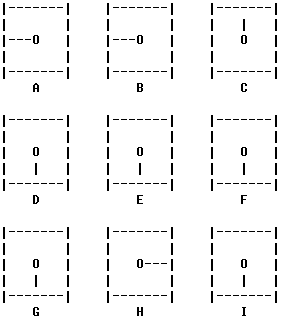/ Vijos / 题库 /

# 描述1 ABDE
2 ABC
3 BCEF
5 BDEFH
6 CFI
7 DEGH
8 GHI
9 EFHI

# 格式

## 输入格式

Your program is to read from standard input. Nine numbers give the start positions of the dials. 0=12 o'clock, 1=3 o'clock, 2=6 o'clock, 3=9 o'clock.

## 输出格式

Your program is to write to standard output. Output a shortest sorted sequence of moves (numbers), which returns all the dials to 12 o'clock. You are convinced that the answer is unique.

# 样例1

## 样例输入1

``````3 3 0
2 2 2
2 1 2
``````

## 样例输出1

``````4 5 8 9
``````

# 提示

Description(Figure 1)

There are nine clocks in a 3*3 array (figure 1). The goal is to return all the dials to 12 o'clock with as few moves as possible. There are nine different allowed ways to turn the dials on the clocks. Each such way is called a move. Select for each move a number 1 to 9. That number will turn the dials 90' (degrees) clockwise on those clocks which are affected according to figure 2 below.

Move Affected clocks

1 ABDE
2 ABC
3 BCEF
5 BDEFH
6 CFI
7 DEGH
8 GHI
9 EFHI

(Figure 2)

Input
Your program is to read from standard input. Nine numbers give the start positions of the dials. 0=12 o'clock, 1=3 o'clock, 2=6 o'clock, 3=9 o'clock.

Output
Your program is to write to standard output. Output a shortest sorted sequence of moves (numbers), which returns all the dials to 12 o'clock. You are convinced that the answer is unique.

# 来源

Vivian Snow
IOI 1994 The Clocks, From PKU 1166

ID
1016

5

4779

1599

33%

15